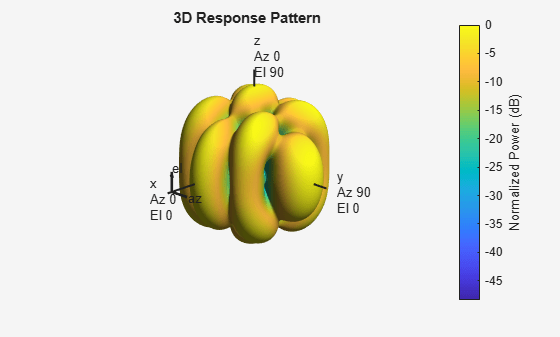Conformal Array

Support for Arrays with Custom Geometry

The phased.ConformalArray object lets you model a phased array with arbitrary geometry. For example, you can use phased.ConformalArray to design:

• A planar array with a nonrectangular geometry, such as a circular array

• An array with nonuniform geometry, such as a linear array with variable spacing

• A nonplanar array

When you use phased.ConformalArray, you must specify these aspects of the array:

• Sensor element of the array

• Element positions

• Direction normal to each array element

Create Default Conformal Array

To create a conformal array with default properties, use this command:

array = phased.ConformalArray
array =
phased.ConformalArray with properties:

Element: [1x1 phased.IsotropicAntennaElement]
ElementPosition: [3x1 double]
ElementNormal: [2x1 double]
Taper: 1

This default conformal array consists of a single phased.IsotropicAntennaElement antenna located at the origin of the local coordinate system. The direction normal to the sensor element is 0° azimuth and 0° elevation.

Uniform Circular Array Created from Conformal Array

This example shows how to construct a 60-element uniform circular array. In constructing a uniform circular array, you can use either the phased.UCA or the phased.ConformalArray System objects. The conformal array approach is more general because it allows you to point the array elements in arbitrary directions. A UCA restricts the array element directions to lie in the plane of the array. This example illustrates how you can use the phased.ConformalArray System object to create any other array shape. Assume an operating frequency of 400 MHz. Tune the array by specifying the arclength between the elements to be 0.5$\lambda$ where $\lambda$ is the wavelength corresponding to the operating frequency. Array elements lie in the x-y-plane. Element normal directions are set to $\left({\varphi }_{n},0\right)$ where ${\varphi }_{n}$ is the azimuth angle of the ${n}^{th}$ array element.

Set the number of elements and the operating frequency of the array.

N = 60;
fc = 400e6;

Compute the element spacing in radians.

theta = 360/N;

Choose the radius so that the inter-element arclength is one-half wavelength.

arclength = 0.5*(physconst('LightSpeed')/fc);

Compute the element azimuth angles. Azimuth angles must lie in the range $\left(-18{0}^{\circ },18{0}^{\circ }\right)$.

ang = (0:N-1)*theta;
ang(ang >= 180.0) = ang(ang >= 180.0) - 360.0;
array = phased.ConformalArray;
zeros(1,N)];
array.ElementNormal = [ang;zeros(1,N)];

Show the UCA array geometry.

viewArray(array)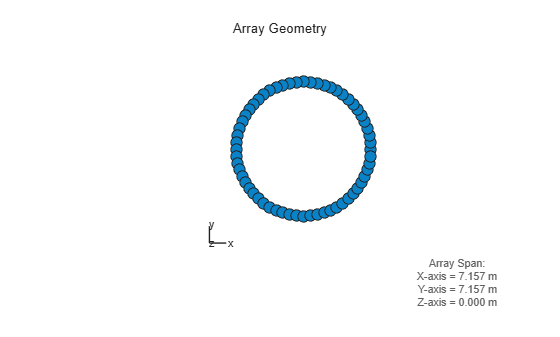Plot the array response pattern at 1 GHz.

pattern(array,1e9,[-180:180],0,'PropagationSpeed',physconst('LightSpeed'),...
'CoordinateSystem','polar','Type','powerdb','Normalize',true)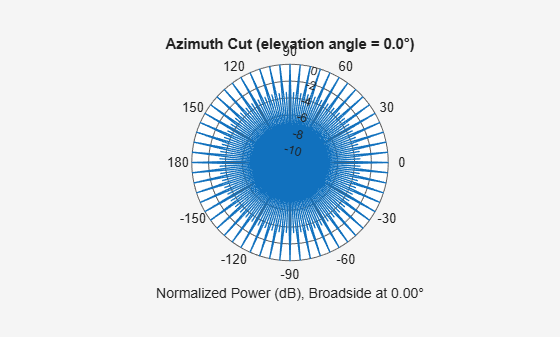Custom Antenna Array

This example shows how to construct and visualize a custom-geometry array containing antenna elements with a custom radiation pattern. The radiation pattern of each element is constant over each azimuth angle and has a cosine pattern for the elevation angles.

Define the custom antenna element and plot its radiation pattern.

az = -180:180;
el = -90:90;
fc = 3e8;
elresp = cosd(el);
antenna = phased.CustomAntennaElement('AzimuthAngles',az,...
'ElevationAngles',el,...
'MagnitudePattern',repmat(elresp',1,numel(az)));
pattern(antenna,3e8,0,el,'CoordinateSystem','polar','Type','powerdb',...
'Normalize',true);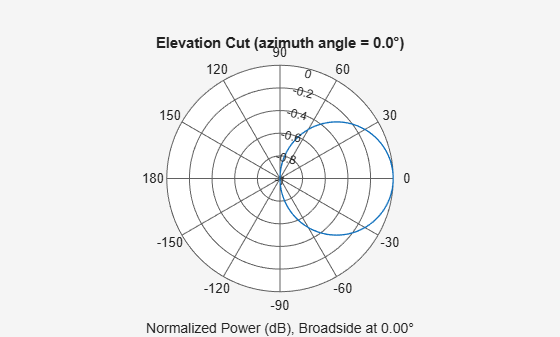Define the locations and normal directions of the elements. All elements lie in the z-plane. The elements are located at (1;0;0) , (0;1;0), and (0;-1;0) meters. The element normal azimuth angles are 0°, 120°, and -120°, respectively. All normal elevation angles are 0°.

xpos = [1 0 0];
ypos = [0 1 -1];
zpos = [0 0 0];
normal_az = [0 120 -120];
normal_el = [0 0 0];

Define a conformal array with those elements.

array = phased.ConformalArray('Element',antenna,...
'ElementPosition',[xpos; ypos; zpos],...
'ElementNormal',[normal_az; normal_el]);

Plot the positions and normal directions of the elements.

viewArray(array,'ShowNormals',true)
view(0,90)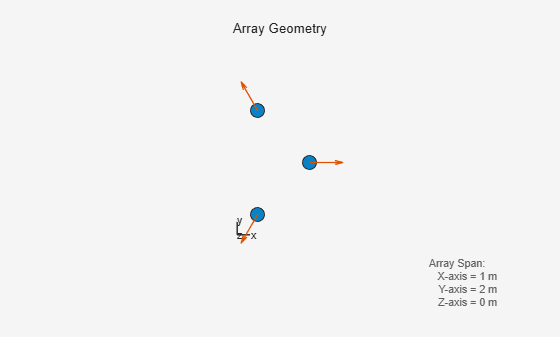pattern(array,fc,az,el,'CoordinateSystem','polar','Type','powerdb',...
'Normalize',true,'PropagationSpeed',physconst('LightSpeed'))